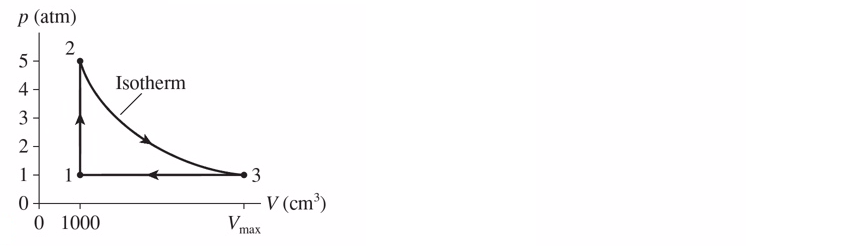# Problem: A heat engine uses 1.3 g of hydrogen and follows the cycle shown in the figure below. a) Find the pressure, volume, and temperature of the hydrogen at points 1, 2 and 3.b) What is the thermal efficiency of this engine?

###### Problem Details

A heat engine uses 1.3 g of hydrogen and follows the cycle shown in the figure below.

a) Find the pressure, volume, and temperature of the hydrogen at points 1, 2 and 3.

b) What is the thermal efficiency of this engine?